# Texas Go Math Grade 4 Lesson 16.1 Answer Key Units of Time

Refer to our Texas Go Math Grade 4 Answer Key Pdf to score good marks in the exams. Test yourself by practicing the problems from Texas Go Math Grade 4 Lesson 16.1 Answer Key Units of Time.

## Texas Go Math Grade 4 Lesson 16.1 Answer Key Units of Time

Essential Question

How can you use models to compare units of time?
Number line model helped to compare the units of time.

Unlock the Problem

The analog clock below has an hour hand, a minute hand, and a second hand to measure time. The time is 4:30:12.Read Math: Read 4:30:12 as 4:30 and 12 seconds, or 30 minutes and 12 seconds after 4.

There are 60 seconds in a minute and 60 minutes in an hour. The clocks below show the length of a second, a minute, and an hour.Are there more minutes or seconds in one hour?
seconds are more in one hour
Explanation:
1 minute = 60 seconds
1 hour = 60 minutes
60 x 60 = 3600 seconds.
so, seconds are more.

Example 1 How does the size of an hour compare to the size of a second?

Think: Multiply the number of minutes in a hour by the number of seconds in a minute.

There are ___________ minutes in an hour.
There are ___________ seconds in a minute.
60 minutes × ___________ = ___________ seconds
There are ___________ seconds in an hour.
So, 1 hour is ___________ times as long as 1 second.
There are 60 minutes in an hour.
There are 60 seconds in a minute.
60 minutes × 60 = 3600 seconds
There are 3600 seconds in an hour.
So, 1 hour is 3600 times as long as 1 second.

Math Talk

Mathematical Processes
How many full turns clock wise does a minute hand make in 3 hours? Explain.
Explanation:
1 hour = 60 full turns
3 x 60 = 180 full turns.

Example 2 Compare measures.

Materials
color pencils

The number line below shows the relationship between days and weeks.

STEP 1 Use a color pencil to shade 1 week on the number line.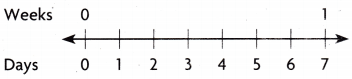Explanation:
Shaded a week on the number line.

STEP 2 Use a different color pencil to shade 1 day on the number line.STEP 3 Compare the size of 1 week to the size of 1 day.
There are _________ days in _________ week.
So, 1 week is _________ times as long as 1 day.
There are 7 days in 1 week.
So, 1 week is 7 times as long as 1 day.

Share and ShowQuestion 1.
Compare the length of a year to the length of a month. Use a model to help.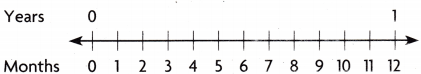1 year is __________ times as long as _________ month.Explanation:
1 year is 12 times as long as 1 month.

Math Talk

Mathematical Processes
Explain how the number line helped you compare the length of a year and the length of a month.
Number line shows the months units and the length of a year covered with month units.

Complete

Question 2.
2 minutes = _________ seconds
Explanation:
1 minute = 60 seconds
60 x 2 = 120 seconds

4 years = ____________ months
Explanation:
1 year = 12 months
4 x 12 = 48 months.

Algebra Compare using <, >, or =.

Question 4.
3 years ____________ 35 months
3 years > 35 months
Explanation:
1 year = 12 months
3 x 12 = 36 months
36 months > 35 months

Question 5.
2 days ____________ 40 hours
2 days  > 40 hours
Explanation:
1 day = 24 hours
2 x 24 = 48 hours
48 hours > 40 hours

Problem Solving

Question 6.
Damien has lived in the apartment building for 5 years. Ken has lived there for 250 weeks. Who has lived in the building longer? Explain. Make a table to help.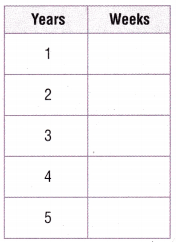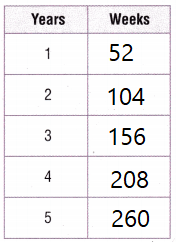Explanation:
Damien has lived in the apartment building for 260 weeks
Ken has lived there for 250 weeks
Damien has lived more

Question 7.H.O.T. Multi-Step How many hours are in a week? Explain.
Answer: A week has 168 hours.
Explanation:
1 day = 24 hours
7 x 24 = 168 hours

Question 8.
Write Math Explain how you know that 9 minutes is less than 600 seconds.
9 minutes is less than 600 seconds.
Explanation:
1 minute = 60 seconds
9 x 60 = 540 seconds
540 < 600

Question 9.
H.O.T. Apply Football practice lasts 3 hours. The coach wants to spend an equal number of minutes on each of 4 different plays. How many minutes will the team spend on each play?
Explanation:
1 hour = 60 minutes.
3 x 60 = 90
90 divided by 4 = 22 minutes on  each play

Martin’s brother just turned 2 years old. What is his brother’s age in months?
Martin’s brother’s age is 24 months.
Explanation:
1 year = 12 months
2 x 12 = 24 months

Question 11.
Multi-Step Mr. Perry drove 2 hours. Mrs. Martin drove 135 minutes. Miss Lawrence drove 25 minutes longer than Mrs. Martin. How much longer did Miss Lawrence drive than Mr. Perry?
40 minutes longer that Miss Lawrence drive than Mr. Perry
Explanation:
Mr. Perry drove 2 hours.
2 x 60 = 120
Mrs. Martin drove 135 minutes.
Miss Lawrence drove 25 minutes longer than Mrs. Martin
135 +25 = 160 minutes.
160 – 120 = 40 minutes.

Question 12.
H.O.T. Shannon’s little brother is 7 weeks old. She is exactly 3 years and 3 days older than he is. How old is Shannon in days? Assume that a year is 365 days.
3 + 7 = 10
3 years 10 days old is Shannon’s little brother
Explanation:
3 x 365 = 1095 + 10 = 1105 days.

Question 13.
Kate’s pet cat is 3 years old. How many months old is her cat?
(A) 12 months
(B) 180 months
(C) 13 months
(D) 36 months
Explanation:
1 year = 12 months
3 x 12 = 36 months

Evaluate Derrick has visited his grandparents for 5 weeks every summer for 6 years. How many days has he visited his grandparents?
(A) 210 days
(B) 42 days
(C) 1,820 days
(D) 420 days
Explanation:
1 week = 7 days
5 x 7 = 35
35 x 6 = 210 days

Question 15.
Multi-Step Damian wants to study for 2 hours. He wants to spend an equal amount of minutes studying each of 3 different subjects. How many minutes will he spend studying each subject?
(A) 120 minutes
(B) 30 minutes
(C) 40 minutes
(D) 180 minutes
Explanation: 1 hour = 60 minutes
2 hours=60 x 2 = 120 minutes
120 divided by 3 = 40 minutes

TEXAS Test Prep

Question 16.
In 7 days, 4 hours and 35 minutes, Michael is flying to Houston. How many minutes is that?
(A) 172 minutes
(B) 10,355 minutes .
(C) 207 minutes
(D) 2,760 minutes
Explanation:
1 hour=60 min
1 day=24hours
4hourus=240 minutes
7 days =168 hours
168 x 60=10080 minutes
10080+275=10355 minutes

### Texas Go Math Grade 4 Lesson 16.1 Homework and Practice Answer Key

Complete.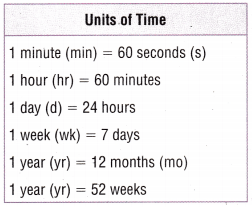Question 1.
9 weeks = ___________ days

Question 2.
4 years = ___________ months

Question 3.
5 minutes = ___________ seconds

___________ hours = 3 days

Compare using <, >, or =.

Question 5.
50 hours ________ 2 days
Explanation:
2 days = 48 hours
50>48

Question 6.
40 days ________ 5 weeks
Explanation:
5weeks=35 days
40 days > 35 days

Question 7.
3 minutes ________ 200 seconds
Explanation:
3 minutes=180 seconds
180<200

Question 8.
8 hours ________ 480 minutes
Explanation:
8 hours=480 minutes
480=480

Problem Solving

Question 9.
Multi-Step It takes Emily 40 minutes to drive to a football game. It takes Mark 1 hour and 15 minutes longer. How many minutes does it take Mark to drive to the football game? Explain.
Explanation:
1 hour = 60 minutes
60+15=75 minutes.

Brandon was on vacation for 2 weeks and 1 day. He spent the same amount of time with each of his 3 brothers. How many days did he spend with each brother? Explain.
Explanation:
2 weeks = 14 days
14+1=15 days.

Lesson Check

Question 11.
Alison’s solar panels have a 10-year guarantee For how many months is the guarantee good?
(A) 60 months
(B) 120 months
(C) 600 months
(D) 520 months
Explanation:
1 year = 12 months
12 x 10= 120 months.

Question 12.
The circus stayed in Bloomington for 3 weeks. How many days was the circus in town?
(A) 21 days
(B) 90 days
(C) 36 days
(D) 72 days
Explanation:
1 week = 7 days
3 x 7 = 21 days.

Question 13.
Multi-Step Carmen takes 1 breath every 4 seconds. How many breaths will she take in 1 minute?
(A) 40
(B) 28
(C) 15
(D) 20
Explanation:
1 minute=60 seconds
60 divided by 4 =15 seconds.

Question 14.
Multi-Step It takes Alden 20 minutes to walk 1 mile. How many miles can Alden walk in 2 hours?
(A) 3 miles
(B) 12 miles
(C) 8 miles
(D) 6 miles
Explanation: 20 x 3 = 60 min
3 x 2 = 6 miles

Multi-Step Greg spent 3 hours practicing his guitar. He spent the same amount of time practicing each of 4 songs. How many minutes did Greg spend practicing each song?
(A) 45 minutes
(B) 30 minutes
(C) 55 minutes
(D) 10 minutes
Explanation:
60 x 3= 180 minutes
180 divided by 4 =45 minutes

Question 16.
Multi-Step This summer, Nora spent 8 days visiting her aunt and 2 weeks visiting her grandmother. In all, how many days did she spend visiting?
(A) 10 days
(B) 22 days
(C) 32 days
(D) 14 days